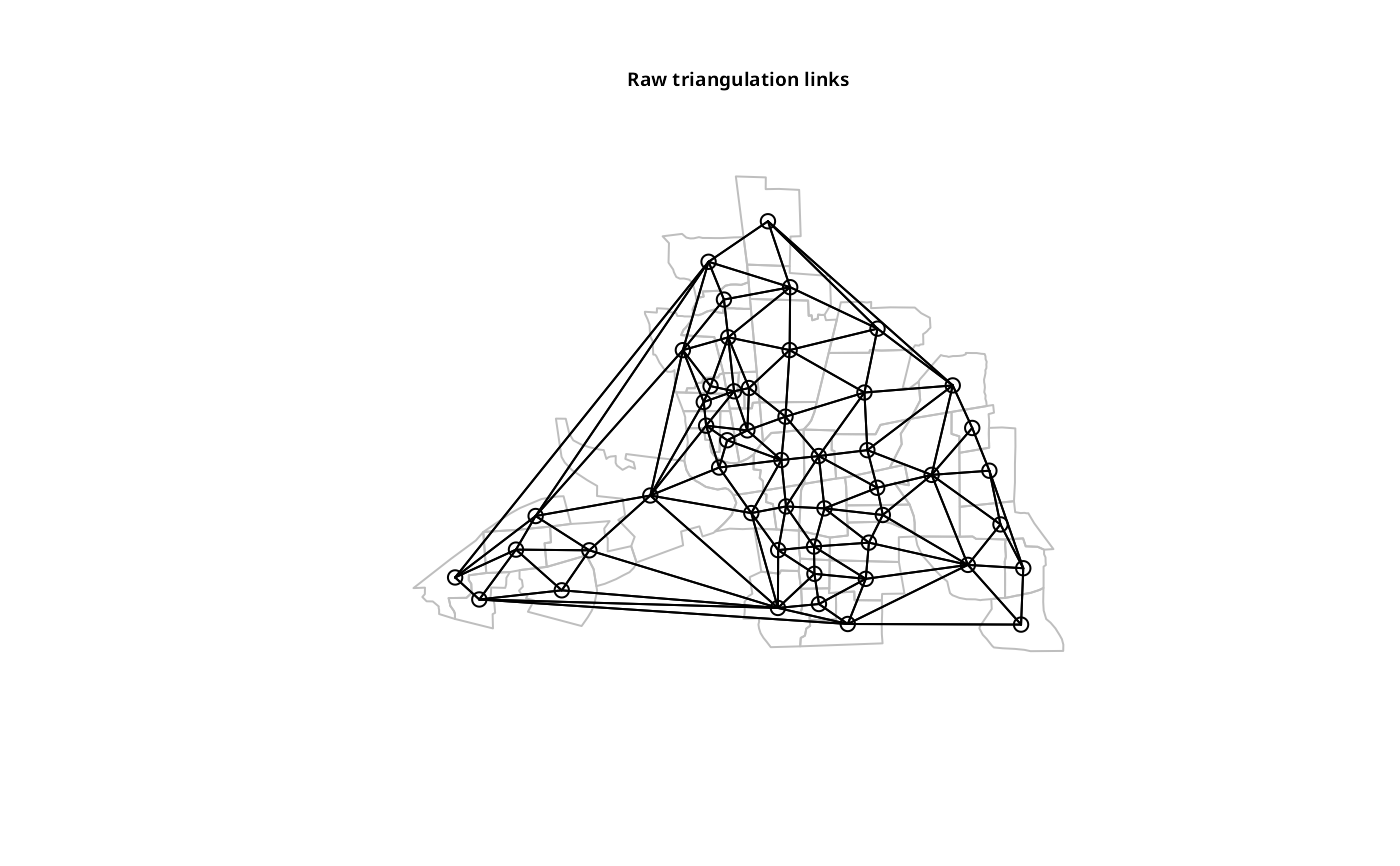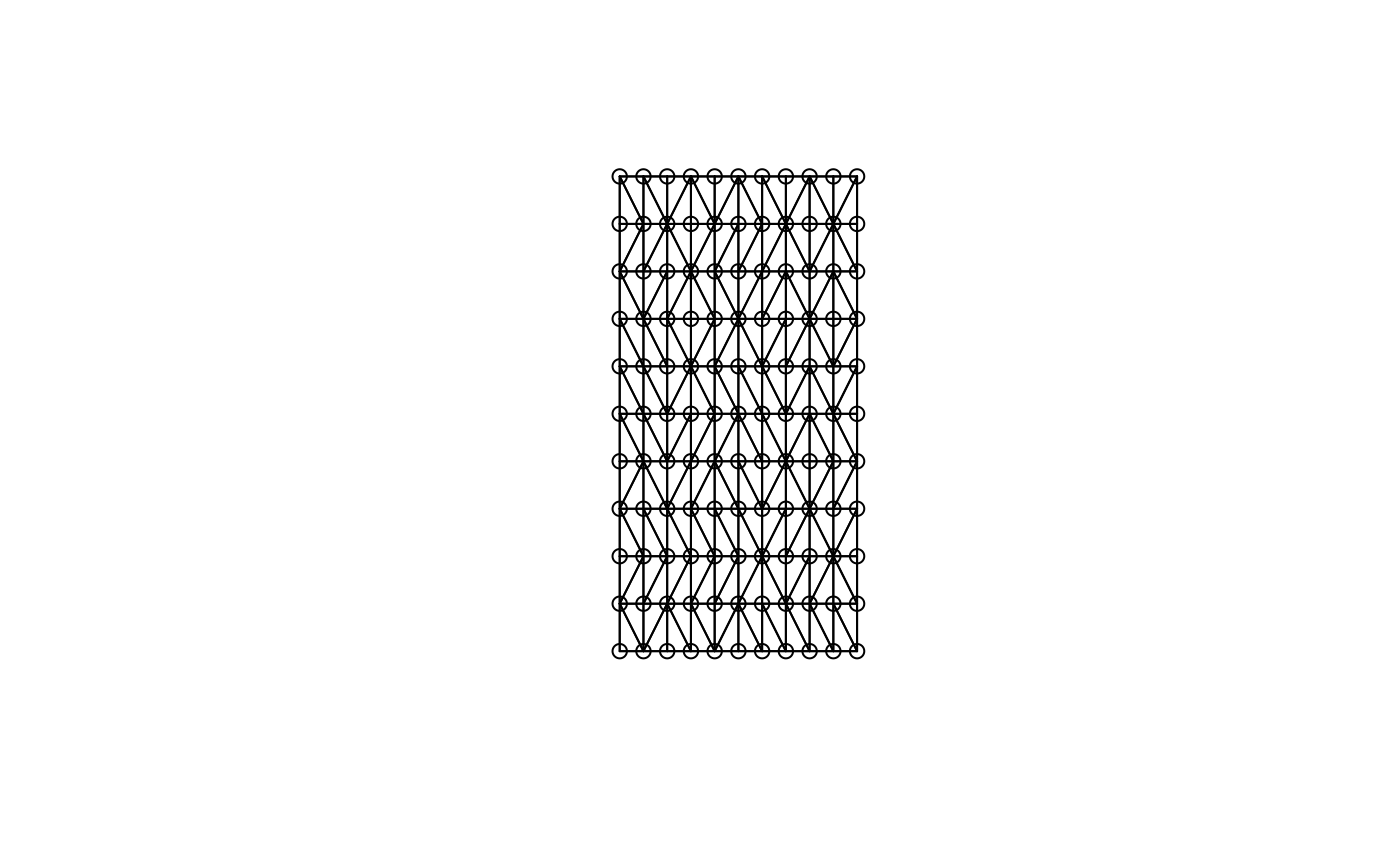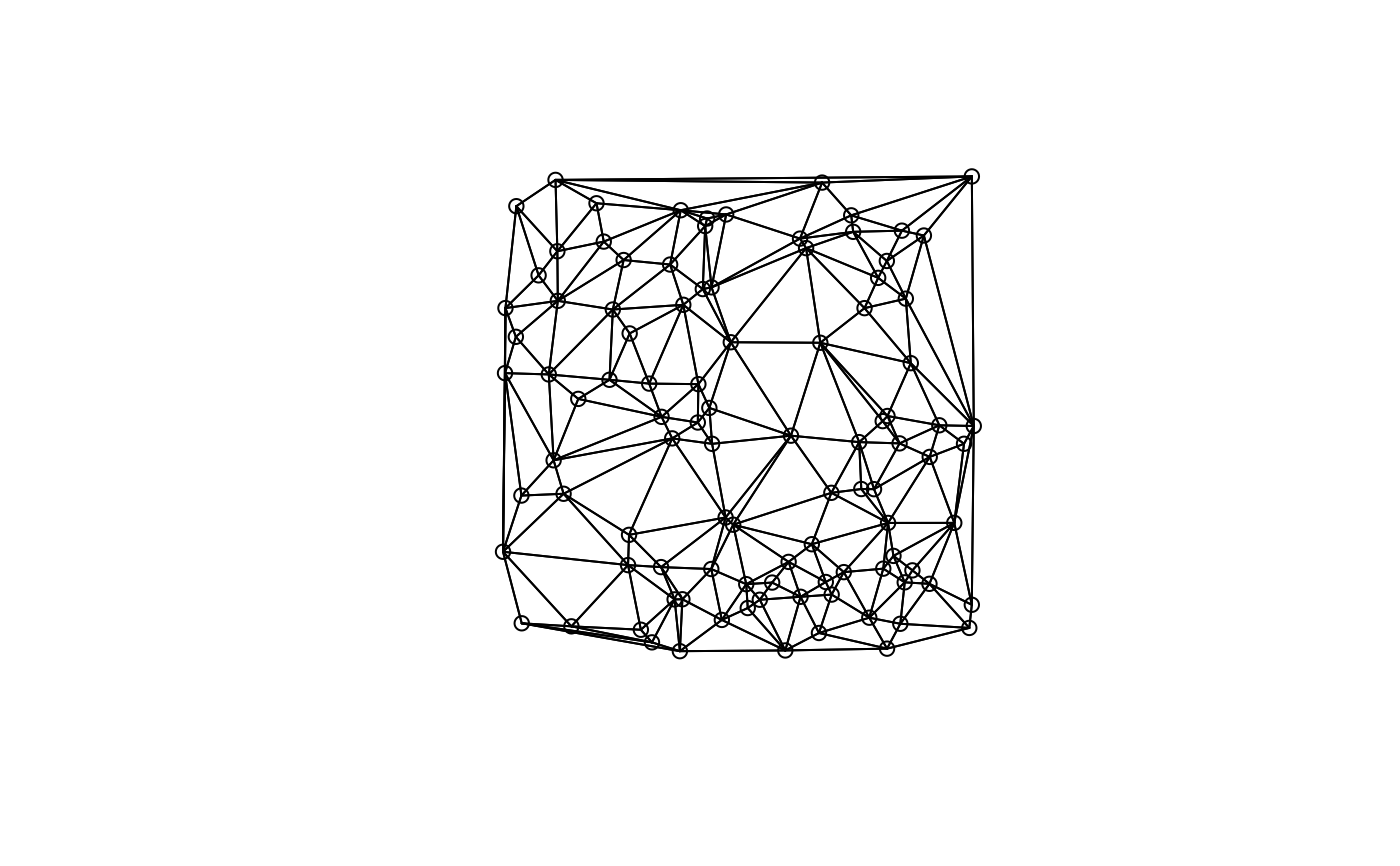The function uses the deldir package to convert a matrix of two-dimensional coordinates into a neighbours list of class nb with a list of integer vectors containing neighbour region number ids.

tri2nb(coords, row.names = NULL)

## Arguments

coords

matrix, data.frame or tibble of point coordinates with two columns, a SpatialPoints object or an sfc points object

row.names

character vector of region ids to be added to the neighbours list as attribute region.id, default seq(1, nrow(x))

## Details

If coordinates are duplicated, this function cannot be used. If the coordinates are from a grid, then they need to be ordered such that the first three are not collinear, so that the first triangle can be constructed. This can be achieved by randomising the order of the coordinates (possibly several times), and then re-ordering the order of the data to match the new order of the neighbour list - if this fix is used, remember to re-order the row.names argument as well as the coordinates! Please also note that triangulation of grid points will give arbitrary diagonal neighbours, which may not be a sensible outcome, and dnearneigh() may serve better where tri2nb() cannot be used.

## Value

The function returns an object of class nb with a list of integer vectors containing neighbour region number ids.

## Author

Roger Bivand Roger.Bivand@nhh.no

knn2nb, dnearneigh, cell2nb

## Examples

columbus <- st_read(system.file("shapes/columbus.shp", package="spData"), quiet=TRUE)
coords <- st_centroid(st_geometry(columbus), of_largest_polygon=TRUE)
ind <- row.names(columbus)
suppressPackageStartupMessages(require(deldir))
col.tri.nb <- tri2nb(coords, row.names=ind)
plot(st_geometry(columbus), border="grey")x <- seq(0,1,0.1)
y <- seq(0,2,0.2)
xy <- expand.grid(x, y)
try(xy.nb <- tri2nb(xy))
seed <- 1234
xid <- sample(1:nrow(xy))
xy.nb <- tri2nb(xy[xid,])
plot(xy.nb, xy[xid,])